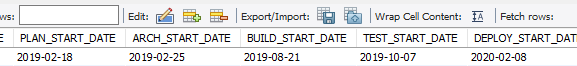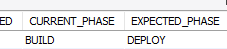troubleshooting Question

# How do I incorporate this CASE into my SELECTasked on
MySQL Server
Here's my current SELECT:

``````select RID from tasks_accomplishments where CREATED_DATE <='{\$asof}'
and  t.PROJECT IN (select DISTINCT t.PROJECT
JOIN resources r ON t.PROJECT=r.PROJID
JOIN projects p ON p.ID=t.PROJECT
where r.USER_ID={\$user_id}
and p.ACTIVE=1))
``````
The problem is the very first line. I can't use CREATED_DATE. Instead, I have to use one of four values:I'm going to use the value that's defined by the value in the EXPECTED_PHASE column......which in this case is DEPLOY. So, I need go grab "2020-02-08."

How?

I wrote this as part of another SELECT:

case when (SELECT EXPECTED_PHASE FROM task_metrics where RID = t.ID) = 'DEPLOY'
then (select DEPLOY_START_DATE from task_metrics where RID = t.ID)
when (SELECT EXPECTED_PHASE FROM task_metrics where RID = t.ID) = 'BUILD'
then (select BUILD_START_DATE from task_metrics where RID = t.ID)
when (SELECT EXPECTED_PHASE FROM task_metrics where RID = t.ID) = 'DEPLOY'
then (select DEPLOY_START_DATE from task_metrics where RID = t.ID)
when (SELECT EXPECTED_PHASE FROM task_metrics where RID = t.ID) = 'ARCH'
then (select ARCH_START_DATE from task_metrics where RID = t.ID)
when (SELECT EXPECTED_PHASE FROM task_metrics where RID = t.ID) = 'TEST'
then (select TEST_START_DATE from task_metrics where RID = t.ID)

...but how would I incorporate that into the SELECT that I've got above?

Hit me!

###### Learn from the best

Network and collaborate with thousands of CTOs, CISOs, and IT Pros rooting for you and your success.

Andrew Hancock - VMware vExpert
See if this solution works for you by signing up for a 7 day free trial.